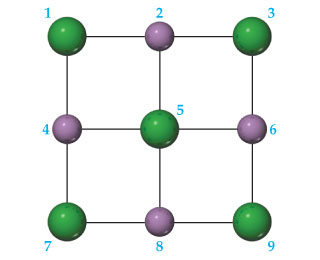Problem: A portion of a two-dimensional "slab" of NaCl(s) is shown here in which the ions are numbered.Consider ion 5. How many repulsive interactions are shown for it?

FREE Expert Solution

We have to determine how many repulsive interactions are shown for ion 5 in the given diagram.

We will start by the fact that opposite charges attract each other, and same charges repel each other.

In the given diagram, the sodium ions (Na+) are shown by purple spheres and the chloride ions (Cl-) are shown by green spheres.

80% (175 ratings)
Problem Details
A portion of a two-dimensional "slab" of NaCl(s) is shown here in which the ions are numbered.Consider ion 5. How many repulsive interactions are shown for it?